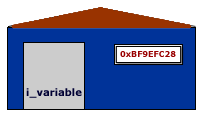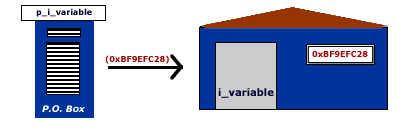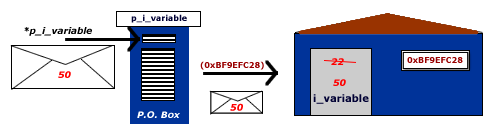# Pointers in C/C++

When programming in C and/or C++, you will encounter the use of pointers.
We are going to begin first doing a review of regular pointers and then we will see how to create and use function pointers.

In C/C++, when we create a static or dynamic variable, this variable will have an address in memory. For example:

If we write the following code:

`int integer_variable;`

An space in memory of the integer size (16-bits, 32-bits, or 64-bits depending the system) will be reserved.

When we assign a value to this variable, this value is store in the memory as a binary number that is represented base on the variable’s type.

```int integer_variable = 4;
integer_variable = 5; /* Change value */```

If we wish to know in which address is this value store we use ‘&’ in front of the variable.

```int main(int argc, char* argv[]){
int i_variable = 22;
printf('i_variable has value %d at address 0x%X\n', i_variable, (unsigned int) &i_variable);
return 0;
}```

First note that we are using a downcasting “(unsigned int)” since there are no negative values in memory address else you will receive a warning from the gcc compiler. Second, in this example we are using %X to indicate printf to represent the address as an hexadecimal value.

This will give us:

`i_variable has value 22 at address 0xBF9EFC28`

If this would be a house for example, i_variable would be the name of the resident while the memory address would be the address of the house.When creating a pointer in C/C++, we are declaring a variable (which have its own address) that will hold an address.

`int* p_i_variable;`

And we instantiate this pointer variable with the address of another variable, in this example would be i_variable.

`int* p_i_variable = &i_variable;`

or you could instantiate in this way:

```int* p_i_variable;
p_i_variable = &i_variable;```

So, we could say that the pointer p_i_variable is like a P. O. Box which many home-owners use when having companies. The P. O. Box have an address but the content of it is pointed to the real address of the owner.If we want to ask where the p_i_variable (P.O. Box) is “pointing at” we can do:

```int main(int argc, char* argv[]){
int i_variable = 22;
int* p_i_variable = &i_variable;
printf('i_variable with value %d has address 0x%X \n', i_variable, (unsigned int) &i_variable);
(unsigned int) &p_i_variable,
(unsigned int) p_i_variable);
}```

You may notice that the first parameter is (unsigned int) &p_i_variable, this is to display the address of the pointer variable, while (unsigned int) p_i_variable is to display the value store in that pointer variable which would be the address of i_variable. Here is an example of the output:

```i_variable with value 22 has address 0xBF877448

Lets say that want to change the value stored in i_variable. You could changed the value directly by using the variable name:

`i_variable = 50;`

Or you could do it indirectly by using the pointer:

`*p_i_variable = 50;`

First notice that we are using the asterisk ‘*’ before the name of the pointer. This tells the compiler the following: To the variable that have the address stored in p_i_variable, we wish to change the value to 50;For example:

```int main(int argc, char* argv[]){
int i_variable = 22;
int* p_i_variable = &i_variable;
printf('i_variable with value %d has address 0x%X \n', i_variable, (unsigned int) &i_variable);
(unsigned int) &p_i_variable,
(unsigned int) p_i_variable);

/* Change value of i_variable directly */
i_variable = 50;
printf('i_variable with value %d has address 0x%X \n', i_variable, (unsigned int) &i_variable);

/* Change value of i_variable indirectly using the pointer p_i_variable */
*p_i_variable = 100;
(unsigned int) &p_i_variable,
(unsigned int) p_i_variable);
printf('i_variable with value %d has address 0x%X \n', i_variable, (unsigned int) &i_variable);
printf('Obtain value of i_variable using pointer p_i_variable: %d\n', *p_i_variable);

return 0;
}```

This would show:

```i_variable with value 22 has address 0xBF982BC8
i_variable with value 50 has address 0xBF982BC8
i_variable with value 100 has address 0xBF982BC8
Obtain value of i_variable using pointer p_i_variable: 100```

One of the things you must have in consideration is the case of dangling pointers. A dangling pointer is a pointer that points to an invalid direction in the memory.

If we create a pointer variable for example:

```/* This is a dangling pointer */
int *pointer_integer;

int integer;

/* Now pointer_integer is not a dangling pointer anymore */
pointer_integer = &integer;
```

and right away we try to read from it, the value inside the variable can be any value, it could be pointing to any direction in memory. If we try to write anything to that direction we could create a segmentation fault.

So if we are not going to use this variable right away, it is a good idea to instantiated with NULL (which have a value of 0):

```/* this is not a dangling pointer */
int *pointer_integer = NULL;```

The next post, we are going to talk about pointers with arrays, and double-pointers.